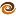EmptyCrate

About Me | Contact Me | Training |ChaiScript.com | YouTube Channel

The second alpha of the Minnow programming language was released recently. Minnow is a new programming language designed around the idea of actors and using a new datastructure building paradigm called ligomorphism.

While programming in Minnow every object is an actor which behaves like it is an independent thread. An actor may or may not actually be tied to an operating system thread at any given moment depending on how many CPU cores you have available. By managing its own notion of timeslices it is able to not overload the OS with too many threads while simultaneously letting you take advantage of every hardware thread available. All function calls between actors are asynchronous messages. Also, the programming language compiles to C, which then compiles to native code, so there is very little runtime overhead.

Using minnow (SVN, and working with the author) I was able to put together the following bit of code which generates a rendering of the mandelbrot fractal set.

The magic with this code, and Minnow itself, is that the code below can (in theory) automatically take advantage of up to 17 CPU’s because of how the work is divided in to images pieces. All without any threading constructs in the code, no locking, no thread safe queues, etc.

Of course, the system is still young and some bugs are still being worked out.

(Full disclosure: The author of minnow and I are cousins)

extern def log(x : double) : double  // Make the c library log and abs functions available
extern def fabs(x : double) : double
feature Color
r : double
g : double
b : double

def Color(t_r:double, t_g:double, t_b:double)
r = t_r
g = t_g
b = t_b
end

def Color()
r = (0.0)
g = (0.0)
b = (0.0)
end

end

feature Point
x : int
y : int

def Point(t_x:int, t_y:int)
x = t_x
y = t_y
end
end

feature Pixel
color : Color
point : Point

def Pixel(t_point:Point, t_color:Color)
color = t_color
point = t_point
end
end

//technically this should be an 'isolated actor' because of the use of SDL.delay
isolated actor SDLWriter

m_width : int
m_height : int

m_pixels : int
screen : SDL.Surface
surface : SDL.Surface

def SDLWriter(t_width:int, t_height:int)
m_width = t_width
m_height = t_height

m_pixels = 0

screen = new SDL.Surface()
surface = new SDL.Surface()

screen.create_main_window(t_width, t_height, 32)
surface.create_surface(t_width, t_height, 32)
end

surface.draw_pixel((p.color.r * 255.0).to_int(), (p.color.g * 255.0).to_int(), (p.color.b * 255.0).to_int(), p.point.x, p.point.y)

m_pixels += 1
SDL.clear_events()

if ((m_pixels/32)*32 == m_pixels)
screen.blit_surface(surface)
end

if (m_pixels == (m_width * m_height))
screen.blit_surface(surface)
while(true)
SDL.delay(100)
SDL.clear_events()
end
end
end
end

actor PixelCalc
m_tl : Point
m_br : Point
m_sdl : SDLWriter
m_width : int
m_height : int
m_scale : double
m_x : double
m_y : double

def PixelCalc(tl:Point, br:Point, sdl:SDLWriter, t_width:int, t_height:int)
m_tl = tl
m_br = br
m_sdl = sdl
m_width = t_width
m_height = t_height
m_x = 0.001643721971153
m_y = -0.822467633298876
m_scale = 0.0000000001
end

// This is where the color value of a particular pixel in the set is generated
def GetColor(xcoord:int, ycoord:int) : Color
xscaled:double = xcoord.to_double()/(m_width.to_double()/m_scale) + (m_x - (m_scale/2.0 ))
yscaled:double = ycoord.to_double()/(m_height.to_double()/m_scale) + (m_y - (m_scale/2.0 ))

x:double = xscaled
y:double = yscaled

iteration:int = 0
max_iteration:int = 2000

stop_iteration:int = max_iteration

while ( iteration < stop_iteration )
if ((x*x) + (y*y) > (2.0*2.0) && stop_iteration == max_iteration)

stop_iteration = iteration + 5
end

xtemp:double = (x*x) - (y*y) + xscaled
y = (2.0*x*y) + yscaled
x = xtemp
iteration += 1
end

if (iteration == max_iteration)
return new Color(0.0,0.0,0.0)
else
value:double = ((iteration + 1).to_double() - (log(log(fabs(x * y))))/log(2.0))
red:double = 0.0
green:double = 0.0
blue:double = 0.0

colorval:int = (value * 10.0).to_int()

if (colorval < 256)
red = (colorval.to_double())/256.0
else
colorband:int = ((colorval - 256) - (((colorval - 256) / 1024) * 1024))/256
mod256:int = colorval - ((colorval / 256) * 256)
if (colorband == 0)
red = 1.0
green = mod256.to_double() / 255.0
blue = 0.0
elseif (colorband == 1)
red = 1.0
green = 1.0
blue = mod256.to_double() / 255.0
elseif (colorband == 2)
red = 1.0
green = 1.0
blue = 256.0 - (mod256.to_double()/255.0)
else
red = 1.0
green = 256.0 - (mod256.to_double()/255.0)
blue = 0.0
end
end

return new Color(red, green, blue)
end

end

action calc()
y:int = m_tl.y
while (y < m_br.y)
x:int = m_tl.x
while (x < m_br.x)
x += 1
end
y += 1
end
end
end

action main(args : Array[string])
w:int = 512
h:int = 512
sdlwriter:var = spawn SDLWriter(w, h)
calcs:var = new Array[PixelCalc]
x:int = 0
y:int = 0

div:int = 4

while (x < w)
y = 0
while (y < h)
p:var = spawn PixelCalc(new Point(x, y), new Point(x+(w/div), y+(h/div)), sdlwriter, w, h)
p::calc()
calcs.push(p)
y += h/div
end
x += w/div
end
end
My Videos

Related